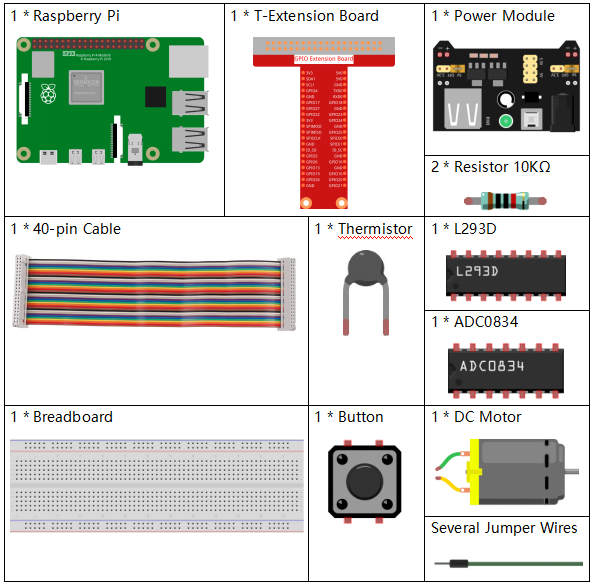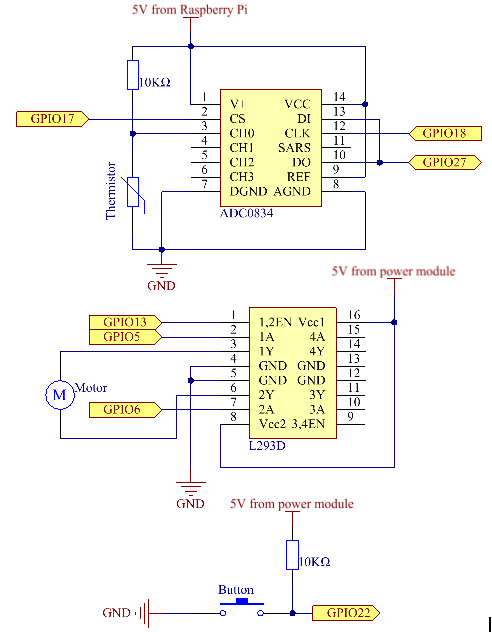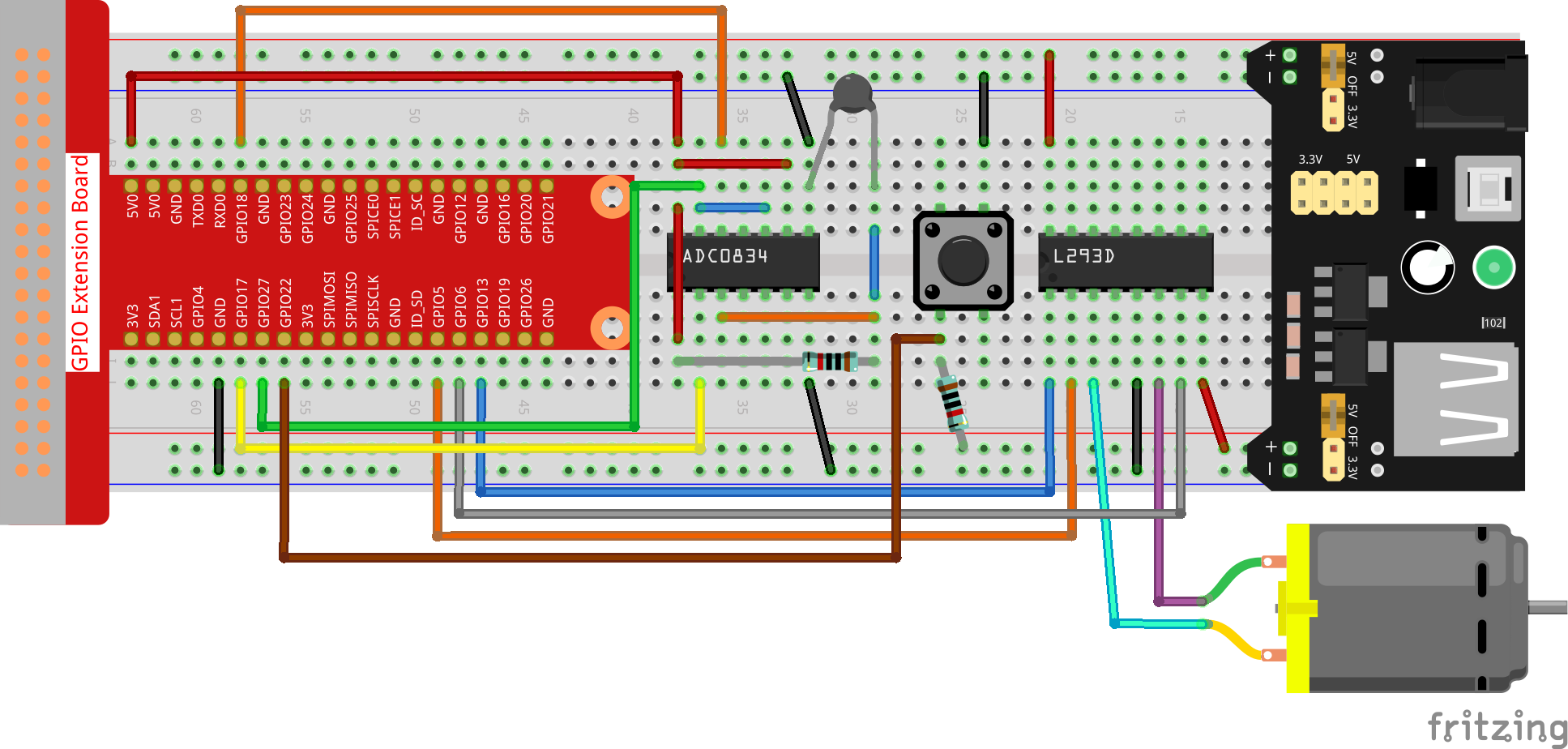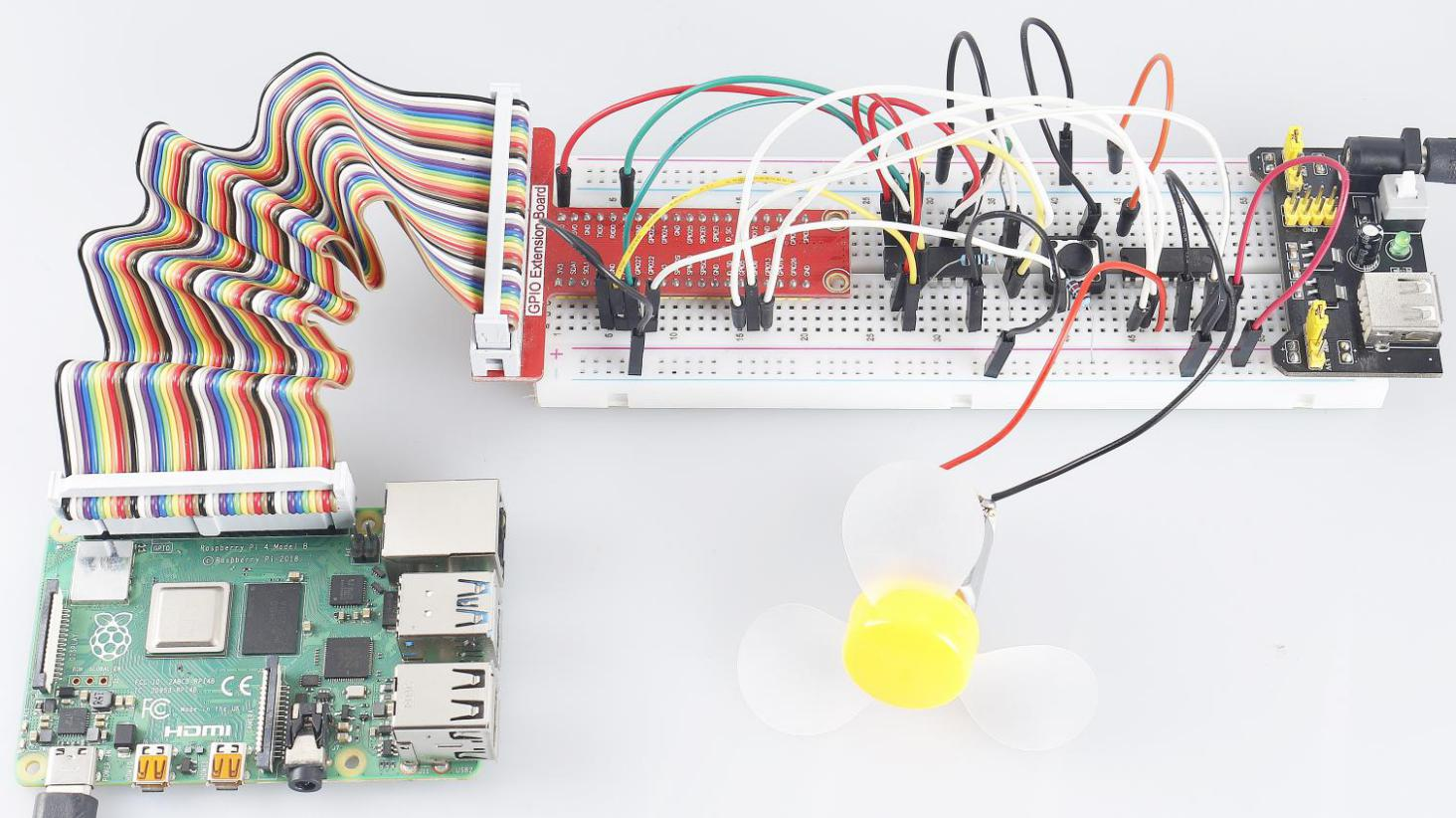# 3.1.4 Smart Fan¶

## Introduction¶

In this course, we will use motors, buttons and thermistors to make a manual + automatic smart fan whose wind speed is adjustable.

## Components¶## Schematic Diagram¶

 T-Board Name physical wiringPi BCM GPIO17 Pin 11 0 17 GPIO18 Pin 12 1 18 GPIO27 Pin 13 2 27 GPIO22 Pin 15 3 22 GPIO5 Pin 29 21 5 GPIO6 Pin 31 22 6 GPIO13 Pin 33 23 13## Experimental Procedures¶

Step 1: Build the circuit.Note

The power module can apply a 9V battery with the 9V Battery Buckle in the kit. Insert the jumper cap of the power module into the 5V bus strips of the breadboard.### For C Language Users¶

Step 2: Get into the folder of the code.

```cd ~/davinci-kit-for-raspberry-pi/c/3.1.4/
```

Step 3: Compile.

```gcc 3.1.4_SmartFan.c -lwiringPi -lm
```

Step 4: Run the executable file above.

```sudo ./a.out
```

As the code runs, start the fan by pressing the button. Every time you press, 1 speed grade is adjusted up or down. There are 5 kinds of speed grades: 0~4. When set to the 4th speed grade and you press the button, the fan stops working with a 0 wind speed.

Note

If it does not work after running, or there is an error prompt: "wiringPi.h: No such file or directory", please refer to C code is not working?.

Once the temperature goes up or down for more than 2℃, the speed automatically gets 1-grade faster or slower.

Code Explanation

```int temperture(){
unsigned char analogVal;
double Vr, Rt, temp, cel, Fah;
Vr = 5 * (double)(analogVal) / 255;
Rt = 10000 * (double)(Vr) / (5 - (double)(Vr));
temp = 1 / (((log(Rt/10000)) / 3950)+(1 / (273.15 + 25)));
cel = temp - 273.15;
Fah = cel * 1.8 +32;
int t=cel;
return t;
}
```

Temperture() works by converting thermistor values read by ADC0834 into temperature values. Refer to 2.2.2 Thermistor for more details.

```int motor(int level){
if(level==0){
digitalWrite(MotorEnable,LOW);
return 0;
}
if (level>=4){
level =4;
}
digitalWrite(MotorEnable,HIGH);
softPwmWrite(MotorPin1, level*25);
return level;
}
```

This function controls the rotating speed of the motor. The range of the Level: 0-4 (level 0 stops the working motor). One level adjustment stands for a 25% change of the wind speed.

```int main(void)
{
setup();
int currentState,lastState=0;
int level = 0;
int currentTemp,markTemp=0;
while(1){
currentTemp=temperture();
if (currentTemp<=0){continue;}
if (currentState==1&&lastState==0){
level=(level+1)%5;
markTemp=currentTemp;
delay(500);
}
lastState=currentState;
if (level!=0){
if (currentTemp-markTemp<=-2){
level=level-1;
markTemp=currentTemp;
}
if (currentTemp-markTemp>=2){
level=level+1;
markTemp=currentTemp;
}
}
level=motor(level);
}
return 0;
}
```

The function main() contains the whole program process as shown:

1. Constantly read the button state and the current temperature.

2. Every press makes level+1 and at the same time, the temperature is updated. The Level ranges 1~4.

3. As the fan works ( the level is not 0), the temperature is under detection. A 2℃+ change causes the up and down of the level.

4. The motor changes the rotating speed with the Level.

### For Python Language Users¶

Step 2: Get into the folder of the code.

```cd ~/davinci-kit-for-raspberry-pi/python
```

Step 3: Run.

```sudo python3 3.1.4_SmartFan.py
```

As the code runs, start the fan by pressing the button. Every time you press, 1 speed grade is adjusted up or down. There are 5 kinds of speed grades: 0~4. When set to the 4th speed grade and you press the button, the fan stops working with a 0 wind speed.

Once the temperature goes up or down for more than 2℃, the speed automatically gets 1-grade faster or slower.

Code

Note

You can Modify/Reset/Copy/Run/Stop the code below. But before that, you need to go to source code path like `davinci-kit-for-raspberry-pi/python`.

```import RPi.GPIO as GPIO
import time
import math

# Set up pins
MotorPin1   = 5
MotorPin2   = 6
MotorEnable = 13
BtnPin  = 22

def setup():
global p_M1,p_M2
GPIO.setmode(GPIO.BCM)
GPIO.setup(MotorPin1, GPIO.OUT)
GPIO.setup(MotorPin2, GPIO.OUT)
p_M1=GPIO.PWM(MotorPin1,2000)
p_M2=GPIO.PWM(MotorPin2,2000)
p_M1.start(0)
p_M2.start(0)
GPIO.setup(MotorEnable, GPIO.OUT, initial=GPIO.LOW)
GPIO.setup(BtnPin, GPIO.IN)

def temperature():
Vr = 5 * float(analogVal) / 255
Rt = 10000 * Vr / (5 - Vr)
temp = 1/(((math.log(Rt / 10000)) / 3950) + (1 / (273.15+25)))
Cel = temp - 273.15
Fah = Cel * 1.8 + 32
return Cel

def motor(level):
if level == 0:
GPIO.output(MotorEnable, GPIO.LOW)
return 0
if level>=4:
level = 4
GPIO.output(MotorEnable, GPIO.HIGH)
p_M1.ChangeDutyCycle(level*25)
return level

def main():
lastState=0
level=0
markTemp = temperature()
while True:
currentState =GPIO.input(BtnPin)
currentTemp=temperature()
if currentState == 1 and lastState == 0:
level=(level+1)%5
markTemp = currentTemp
time.sleep(0.5)
lastState=currentState
if level!=0:
if currentTemp-markTemp <= -2:
level = level -1
markTemp=currentTemp
if currentTemp-markTemp >= 2:
level = level +1
markTemp=currentTemp
level = motor(level)

def destroy():
GPIO.output(MotorEnable, GPIO.LOW)
p_M1.stop()
p_M2.stop()
GPIO.cleanup()

if __name__ == '__main__':
setup()
try:
main()
except KeyboardInterrupt:
destroy()
```

Code Explanation

```def temperature():
Vr = 5 * float(analogVal) / 255
Rt = 10000 * Vr / (5 - Vr)
temp = 1/(((math.log(Rt / 10000)) / 3950) + (1 / (273.15+25)))
Cel = temp - 273.15
Fah = Cel * 1.8 + 32
return Cel
```

temperture() works by converting thermistor values read by ADC0834 into temperature values. Refer to 2.2.2 Thermistor for more details.

```def motor(level):
if level == 0:
GPIO.output(MotorEnable, GPIO.LOW)
return 0
if level>=4:
level = 4
GPIO.output(MotorEnable, GPIO.HIGH)
p_M1.ChangeDutyCycle(level*25)
return level
```

This function controls the rotating speed of the motor. The range of the Lever: 0-4 (level 0 stops the working motor). One level adjustment stands for a 25% change of the wind speed.

```def main():
lastState=0
level=0
markTemp = temperature()
while True:
currentState =GPIO.input(BtnPin)
currentTemp=temperature()
if currentState == 1 and lastState == 0:
level=(level+1)%5
markTemp = currentTemp
time.sleep(0.5)
lastState=currentState
if level!=0:
if currentTemp-markTemp <= -2:
level = level -1
markTemp=currentTemp
if currentTemp-markTemp >= 2:
level = level +1
markTemp=currentTemp
level = motor(level)
```

The function main() contains the whole program process as shown:

1. Constantly read the button state and the current temperature.

2. Every press makes level+1 and at the same time, the temperature is updated. The Level ranges 1~4.

3. As the fan works ( the level is not 0), the temperature is under detection. A 2℃+ change causes the up and down of the level.

4. The motor changes the rotating speed with the Level.

## Phenomenon Picture¶